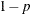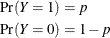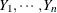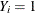# The HPGENSELECT Procedure

### Response Distributions

The response distribution is the probability distribution of the response (target) variable. The HPGENSELECT procedure can fit data for the following distributions:

• binary distribution

• binomial distribution

• gamma distribution

• inverse Gaussian distribution

• multinomial distribution (ordinal and nominal)

• negative binomial distribution

• normal (Gaussian) distribution

• Poisson distribution

• Tweedie distribution

• zero-inflated negative binomial distribution

• zero-inflated Poisson distribution

Expressions for the probability distributions (probability density functions for continuous variables or probability mass functions for discrete variables) are shown in the section Response Probability Distribution Functions. The expressions for the log-likelihood functions of these distributions are given in the section Log-Likelihood Functions.

The binary (or Bernoulli) distribution is the elementary distribution of a discrete random variable that can take on two values that have probabilities p and. Suppose the random variable is denoted Y andThe value that is associated with probability p is often termed the event or "success"; the complementary event is termed the non-event or "failure." A Bernoulli experiment is a random draw from a binary distribution and generates events with probability p.

Ifare n independent Bernoulli random variables, then their sum follows a binomial distribution. In other words, ifdenotes an event (success) in the ith Bernoulli trial, a binomial random variable is the number of events (successes) in n independent Bernoulli trials. If you use the events/trials syntax in the MODEL statement and you specify the DISTRIBUTION=BINOMIAL option, the HPGENSELECT procedure fits the model as if the data had arisen from a binomial distribution. For example, the following statements fit a binomial regression model that has regressors x1 and x2. The variables e and t represent the events and trials, respectively, for the binomial distribution:

proc hpgenselect;
model e/t = x1 x2 / distribution=Binomial;
run;


If the events/trials syntax is used, then both variables must be numeric and the value of the events variable cannot be less than 0 or exceed the value of the trials variable. A "Response Profile" table is not produced for binomial data, because the response variable is not subject to levelization.

The multinomial distribution is a generalization of the binary distribution and allows for more than two outcome categories. Because there are more than two possible outcomes for the multinomial distribution, the terminology of "successes," "failures," "events," and "non-events" no longer applies. For multinomial data, these outcomes are generically referred to as "categories" or levels.

Whenever the HPGENSELECT procedure determines that the response variable is listed in a CLASS statement and has more than two levels (unless the events/trials syntax is used), the procedure fits the model as if the data had arisen from a multinomial distribution. By default, it is then assumed that the response categories are ordered and a cumulative link model is fit by applying the default or specified link function. If the response categories are unordered, then you should fit a generalized logit model by choosing LINK=GLOGIT in the MODEL statement.

If the response variable is not listed in a CLASS statement and a response distribution is not specified in a DISTRIBUTION= option, then a normal distribution that uses the default or specified link function is assumed.